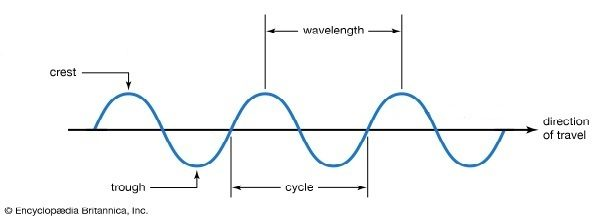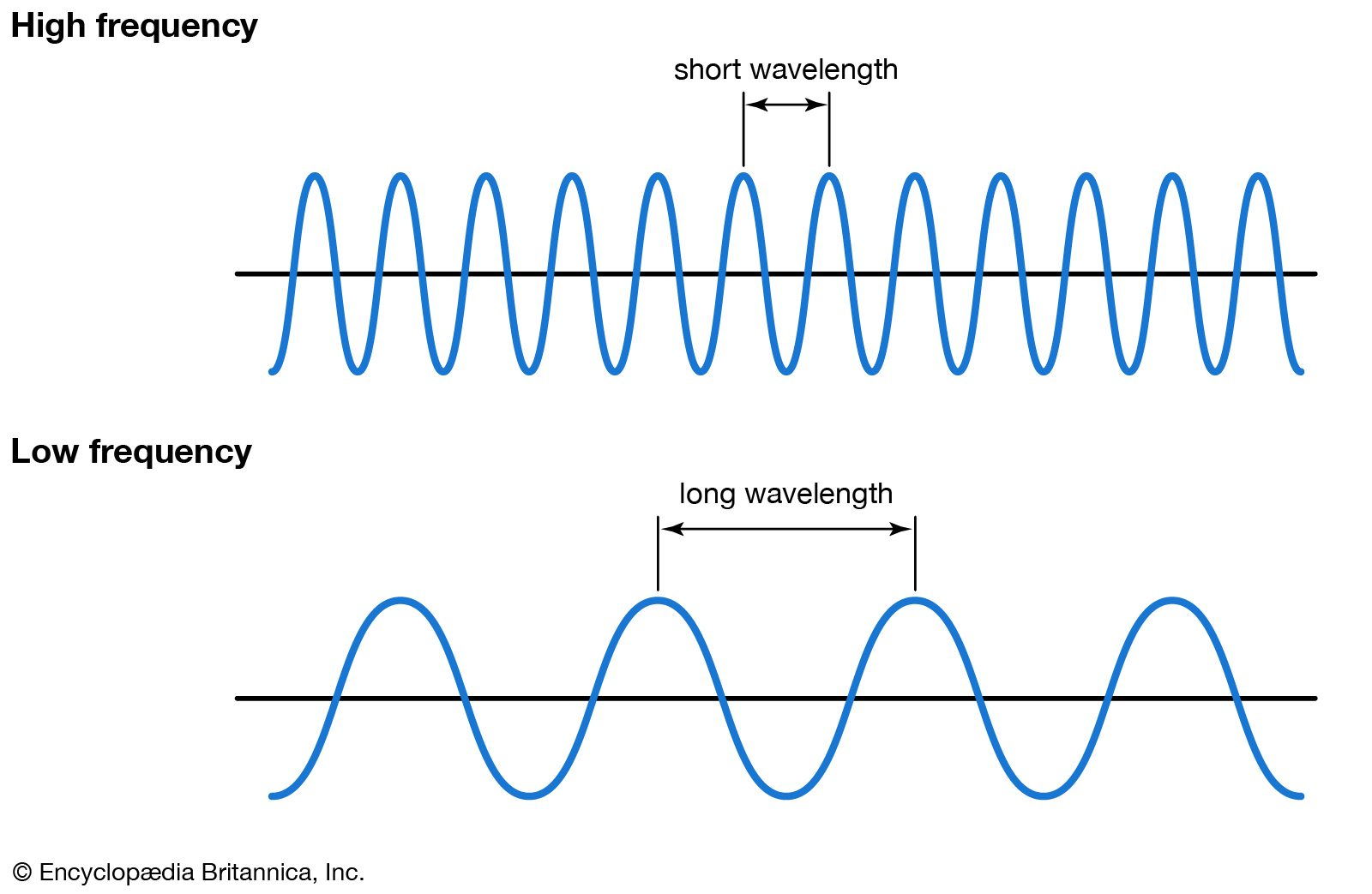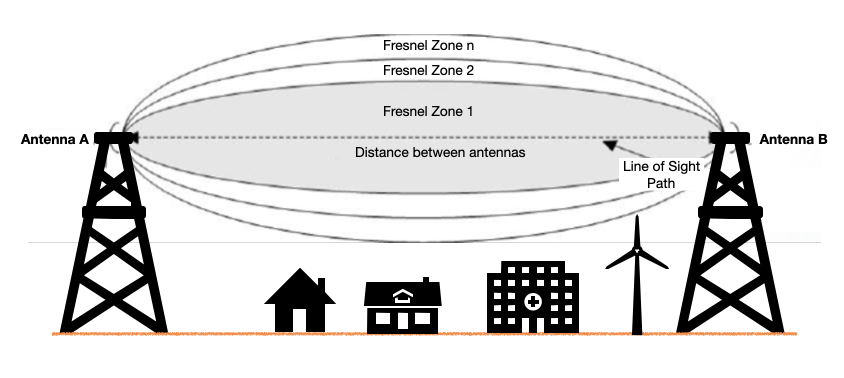# Frequency Calculator

Created by Rita Rain
Reviewed by Dominik Czernia, PhD candidate and Jack Bowater
Last updated: May 05, 2022

The frequency calculator will let you find a wave's frequency given the wavelength and its velocity or period in no time. You can choose a wave velocity from the preset list, so you don't have to remember, e.g., the speed of sound (which you can read more about in our speed of sound calculator).

In the text, you'll also find the frequency definition, two frequency formulas (period to frequency and wavelength to frequency), and a few examples showing how to calculate frequency.

If you want to know more about waves, check the wavelength calculator, and if you want to know the frequency of musical notes, check out the note frequency calculator.

## Frequency definition and the frequency formula

Have a look at the following model of a wave; it will help you understand the terms used in the frequency definition below it.Frequency is the number of completed wave cycles per second. In other words, frequency tells us how many wave crests pass a given point in a second.

This frequency definition leads us to the simplest frequency formula:

f = 1 / T.

f denotes frequency and T stands for the time it takes to complete one wave cycle measured in seconds.

The SI frequency unit is Hertz (Hz), which equals 1/s (one cycle per second). Other frequency units include millihertz (mHz), kilohertz (kHz), megahertz (MHz), gigahertz (GHz), and terahertz (THz).

## Frequency equation from the wavelength

Have a look at another picture which will allow us to see that frequency is connected to wavelength. Wavelength is the distance between two adjacent crests (or troughs). In other words - it is the length of one wave cycle. The longer the wavelength, the lower the frequency:Another fact we need - how fast the waves travel at (wave velocity) determines how many of them will pass a given point per second. This means the higher the wave velocity, the higher the frequency.

These two relationships between frequency and wavelength (λ), and between frequency and velocity (v), bring us to the following frequency equation:

f = v / λ.

## How to use the frequency calculator?

Our frequency calculator incorporates the above-mentioned frequency formulas, therefore you can use it as a period to frequency calculator or a wavelength to frequency calculator.

How to use it as a period to frequency calculator? (How to find frequency if you know period?)

1. Input the time in which one wave cycle occurs (period). The calculator will determine the frequency.

2. You can use this calculator to determine the period, if you know its frequency.

How to use it as a wavelength to frequency calculator? (How to calculate frequency from wavelength?)

1. Enter the wave velocity into the second field or choose the type of wave and its medium from the list in the first field. By default, it is set to light in a vacuum.

2. Enter the wavelength, and the frequency value will appear.

So, basically, you can enter any two variables, and the third will appear immediately 😀

## Example: How to calculate frequency from the period?

To resolve any doubts about how to calculate frequency from the period, let's analyze some simple examples. Firstly, recall the frequency equation:

f = 1 / T.

Example 1:

How to find the frequency of a wave in which one cycle is completed in 0.25s:

f = 1 / 0.25 s

f = 4 * 1/s

Remember to convert "1/s" to the frequency unit:

f = 4 Hz

Example 2:

How to find the frequency of a wave in which 360 cycles occur in 1 minute:

f = 360 / 1 min

f = 360 / 60 s

f = 6/s = 6 Hz

## Example: How to find the frequency of a wave?

This time, we want to learn how to find the frequency of a wave if you're given the wave velocity and wavelength. You need to use the following frequency formula:

f = v / λ.

Example 1:

A wave's velocity equals 320 m/s and its wavelength is 8 m. Find its frequency.

f = 320 m/s / 8 m

f = 40/s

f = 40 Hz

Example 2:

Find the frequency of light if wavelength equals 3000 km.

The wave velocity equals the speed of light in vacuum:

v = c ≈ 300,000 km/s

f ≈ 300,000 km/s / 3000 km

f ≈ 100/s ≈ 100 Hz

f ≈ 100 Hz

## Real-life application

In wireless communication, we have an **ellipsoid volumebetween the transmitter antenna and the receiver antenna**. This region is determined by the distance between the antennas and the frequency of the wireless wave. It is called Fresnel Zone and looks like this:From the main Fresnel zone formula, we infer that the higher the frequency, the smaller the ellipsoid volume required to be free for proper wireless communication. Contrarily, for a low frequency, we get a bigger Fresnel zone which might be easily blocked by buildings or trees, and thus, produce non-estable wireless communication.

Waves with different frequencies give rise to beats and let us calculate beat frequency, while the volume of a chamber greatly influences the Helmholtz resonance frequency.

## FAQ

### How to calculate frequency?

You need to either know the wavelength and the velocity or the wave period (the time it takes to complete one wave cycle). If you know the period:

1. Convert it to seconds if needed and divide 1 by the period.
2. The result will be the frequency expressed in Hertz.

If you want to calculate the frequency from wavelength and wave velocity:

1. Make sure they have the same length unit.
2. Divide the wave velocity by the wavelength.
3. Convert the result to Hertz. 1/s equals 1 Hertz.

### How do I find wavelength from frequency?

1. Determine the wave velocity.
2. Determine the frequency.
3. Convert Hertz to 1/s.
4. Make sure the wave velocity and the frequency have the same time unit.
5. Divide the wave velocity by the frequency.

### What is the relationship between frequency and wavelength?

Frequency equals wave velocity divided by wavelength. Therefore, the longer the wavelength, the lower the frequency, and the shorter the wavelength, the higher the frequency. In other words, frequency is inversely proportional to wavelength.

### What is frequency measured in?

The frequency of a wave is measured in Hertz. 1 Hertz equals 1/s - one cycle per second. The related units include millihertz (one-thousandth of a Hertz), kilohertz (a thousand Hertz), megahertz (a million Hertz), and gigahertz (a billion Hertz). The unit is named after Heinrich Rudolf Hertz, the physicist who proved the existence of electromagnetic waves.

### How to find the frequency of a wave?

1. Determine the wave velocity.
2. Determine the wavelength.
3. Make sure the wave velocity and the wavelength have the same length unit, e.g., if velocity is expressed in meters per second, the wavelength should be expressed in meters.
4. Divide the wave velocity by the wavelength.
5. Convert the result to Hertz. One Hertz equals 1/s - one cycle per second.

### What frequency is 5G?

5G, which is the fifth generation technology standard for cellular networks, operates at various frequency bands which fall into two frequency ranges. Frequency range 1 is from 450 MHz to 6 GHz, and frequency range 2 is from 24.25 GHz to 52.6 GHz.

### Which color has the highest frequency?

Violet is the color with the highest frequency, which ranges from around 670 to 750 terahertz. On the other side of the spectrum there's red with a frequency between 430 and 480 terahertz.

### What is the relationship between frequency and energy?

Energy is directly proportional to frequency. In other words, the higher the frequency, the greater the energy. The relationship between frequency and energy is described by the following formula for the energy of a photon:

E = h ⨉ f

"E" is the symbol for energy, "h" is Planck's constant, and "f" stands for frequency.

### What wave has the highest frequency?

Gamma rays are electromagnetic waves with the highest frequency, that is more than 1019 Hz. They have the highest energy and shortest wavelengths of all electromagnetic waves. Their high energy enables them to detach electrons from atoms and damage living cells. Sources of gamma rays include neutron stars, supernovas, nuclear explosions, and lightning.

### How to get period from frequency?

1. The formula for period is T = 1 / f, where "T" is period – the time it takes for one cycle to complete, and "f" is frequency.
2. To get period from frequency, first convert frequency from Hertz to 1/s.
3. Now divide 1 by the frequency. The result will be time (period) expressed in seconds.
Rita Rain
Wave velocity of
light in vacuum
Wave velocity (v)
m/s
Wavelength (λ)
m
Period (T)
sec
Frequency (f)
Hz
People also viewed…

### Alfvén velocity

The Alfvén velocity calculator helps you compute the velocity of a magnetohydrodynamic wave, a type of a plasma wave.

### Black Hole Collision Calculator

The Black Hole Collision Calculator lets you see the effects of a black hole collision, as well as revealing some of the mysteries of black holes, come on in and enjoy!